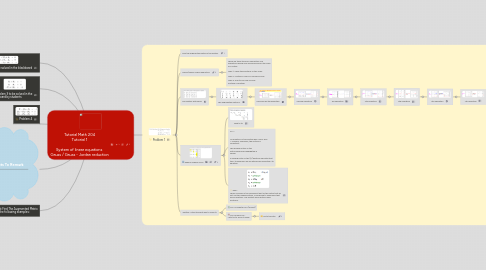# Tutorial Math 204 Tutorial 1 System of linear equations Gauss / Gauss - Jordan reductionRogelio Yoyontzin
Get Started. It's FreeTutorial Math 204 Tutorial 1 System of linear equations Gauss / Gauss - Jordan reduction## 1. Problem 1

### 1.2. Gauss/Gauss Jordan Reduction

1.2.1. There are three types of elementary row operations which may be performed on the rows of a matrix: Type 1: Swap the positions of two rows. Type 2: Multiply a row by a nonzero scalar. Type 3: Add to one row a scalar multiple of another.

### 1.3. The system with zeros

1.3.1. The Augmented Matrix is:

1.3.1.1. Now we use the algorithm

1.3.1.1.1. Second operation

### 1.4. Reduce echalon form

1.4.1. What is it?

1.4.2. So…? No equation of this system has a form zero = nonzero; Therefore, the system is consistent. The leading entries in the matrix have been highlighted in yellow. A leading entry on the (i,j) position indicates that the j-th unknown will be determined using the i-th equation.

1.4.3. Then… Those columns in the coefficient part of the matrix that do not contain leading entries, correspond to unknowns that will be arbitrary. The system has infinitely many solutions:

### 1.5. Is this the best way to solve it?

1.5.1. For a computer YES (almost)

1.5.2. For Humans NO… Let's try to solve it easier.

1.5.2.1. Go to this site: